# jtools

This package consists of a series of functions created by the author (Jacob) to automate otherwise tedious research tasks. At this juncture, the unifying theme is the more efficient presentation of regression analyses. There are a number of functions for visualizing and doing inference for interaction terms. Support for the `survey` package’s `svyglm` objects as well as weighted regressions is a common theme throughout.

Note: This is beta software. Bugs are possible, both in terms of code-breaking errors and more pernicious errors of mistaken computation.

## Installation

For the most stable version, simply install from CRAN.

``install.packages("jtools")``

If you want the latest features and bug fixes then you can download from Github. To do that you will need to have `devtools` installed if you don’t already:

``install.packages("devtools")``

Then install the package from Github.

``devtools::install_github("jacob-long/jtools")``

You should also check out the `dev` branch of this repository for the latest and greatest changes, but also the latest and greatest bugs. To see what features are on the roadmap, check the issues section of the repository, especially the “enhancement” tag.

## Usage

Here’s a synopsis of the current functions in the package:

### Summarizing regressions (`summ`, `plot_summs`, `export_summs`)

`summ` is a replacement for `summary` that provides the user several options for formatting regression summaries. It supports `glm`, `svyglm`, and `merMod` objects as input as well. It supports calculation and reporting of robust standard errors via the `sandwich` package.

Basic use:

``````fit <- lm(mpg ~ hp + wt, data = mtcars)
summ(fit)``````
``````#> MODEL INFO:
#> Observations: 32
#> Dependent Variable: mpg
#> Type: OLS linear regression
#>
#> MODEL FIT:
#> F(2,29) = 69.21, p = 0.00
#> R² = 0.83
#>
#> Standard errors: OLS
#>              Est. S.E. t val.    p
#> (Intercept) 37.23 1.60  23.28 0.00 ***
#> hp          -0.03 0.01  -3.52 0.00  **
#> wt          -3.88 0.63  -6.13 0.00 ***``````

It has several conveniences, like re-fitting your model with scaled variables (`scale = TRUE`). You have the option to leave the outcome variable in its original scale (`transform.response = TRUE`), which is the default for scaled models. I’m a fan of Andrew Gelman’s 2 SD standardization method, so you can specify by how many standard deviations you would like to rescale (`n.sd = 2`).

You can also get variance inflation factors (VIFs) and partial/semipartial (AKA part) correlations. Partial correlations are only available for OLS models. You may also substitute confidence intervals in place of standard errors and you can choose whether to show p values.

``summ(fit, scale = TRUE, vifs = TRUE, part.corr = TRUE, confint = TRUE, pvals = FALSE)``
``````#> MODEL INFO:
#> Observations: 32
#> Dependent Variable: mpg
#> Type: OLS linear regression
#>
#> MODEL FIT:
#> F(2,29) = 69.21, p = 0.00
#> R² = 0.83
#>
#> Standard errors: OLS
#>              Est.  2.5% 97.5% t val.  VIF partial.r part.r
#> (Intercept) 20.09 19.15 21.03  43.82 <NA>      <NA>   <NA>
#> hp          -2.18 -3.44 -0.91  -3.52 1.77     -0.55  -0.27
#> wt          -3.79 -5.06 -2.53  -6.13 1.77     -0.75  -0.47
#>
#> Continuous predictors are mean-centered and scaled by 1 s.d.``````

Cluster-robust standard errors:

``````data("PetersenCL", package = "sandwich")
fit2 <- lm(y ~ x, data = PetersenCL)
summ(fit2, robust = "HC3", cluster = "firm")``````
``````#> MODEL INFO:
#> Observations: 5000
#> Dependent Variable: y
#> Type: OLS linear regression
#>
#> MODEL FIT:
#> F(1,4998) = 1310.74, p = 0.00
#> R² = 0.21
#>
#> Standard errors: Cluster-robust, type = HC3
#>             Est. S.E. t val.    p
#> (Intercept) 0.03 0.07   0.44 0.66
#> x           1.03 0.05  20.36 0.00 ***``````

Of course, `summ` like `summary` is best-suited for interactive use. When it comes to sharing results with others, you want sharper output and probably graphics. `jtools` has some options for that, too.

First, for tabular output, `export_summs` is an interface to the `huxtable` package’s `huxreg` function that preserves the niceties of `summ`, particularly its facilities for robust standard errors and standardization. It also concatenates multiple models into a single table.

``````fit <- lm(mpg ~ hp + wt, data = mtcars)
fit_b <- lm(mpg ~ hp + wt + disp, data = mtcars)
fit_c <- lm(mpg ~ hp + wt + disp + drat, data = mtcars)
coef_names <- c("Horsepower" = "hp", "Weight (tons)" = "wt",
"Displacement" = "disp", "Rear axle ratio" = "drat",
"Constant" = "(Intercept)")
export_summs(fit, fit_b, fit_c, scale = TRUE, transform.response = TRUE, coefs = coef_names)``````
 Model 1 Model 2 Model 3 Horsepower -0.36 ** -0.35 * -0.40 ** (0.10) (0.13) (0.13) Weight (tons) -0.63 *** -0.62 ** -0.56 ** (0.10) (0.17) (0.18) Displacement -0.02 0.08 (0.21) (0.22) Rear axle ratio 0.16 (0.12) Constant 0.00 0.00 0.00 (0.08) (0.08) (0.08) N 32 32 32 R2 0.83 0.83 0.84 *** p < 0.001; ** p < 0.01; * p < 0.05.

In RMarkdown documents, using `export_summs` and the chunk option `results = 'asis'` will give you nice-looking tables in HTML and PDF output. Using the `to.word = TRUE` argument will create a Microsoft Word document with the table in it.

Another way to get a quick gist of your regression analysis is to plot the values of the coefficients and their corresponding uncertainties with `plot_summs` (or the closely related `plot_coefs`). `jtools` has made some slight changes to `ggplot2` geoms to make everything look nice; and like with `export_summs`, you can still get your scaled models and robust standard errors.

``````coef_names <- coef_names[1:4] # Dropping intercept for plots
plot_summs(fit, fit_b, fit_c, scale = TRUE, robust = "HC3", coefs = coef_names)``````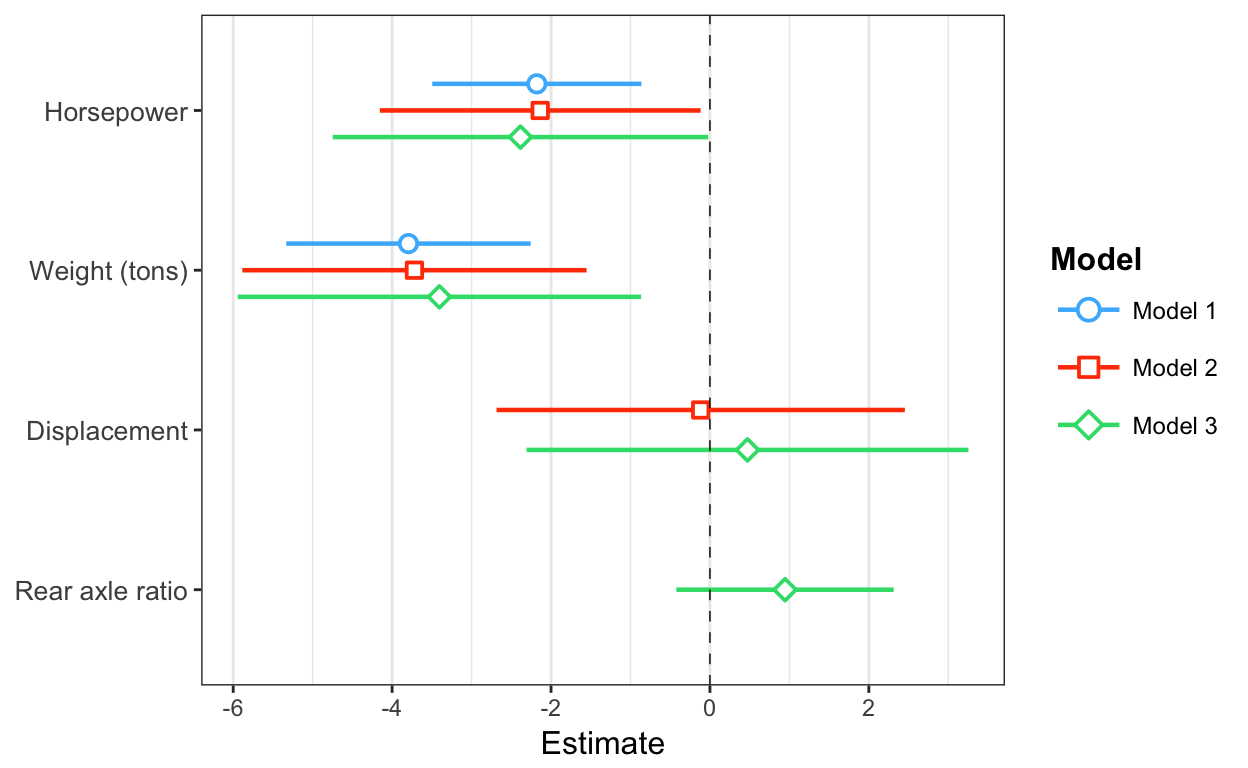And since you get a `ggplot` object in return, you can tweak and theme as you wish.

Another way to visualize the uncertainty of your coefficients is via the `plot.distributions` argument.

``plot_summs(fit_c, scale = TRUE, robust = "HC3", coefs = coef_names, plot.distributions = TRUE)``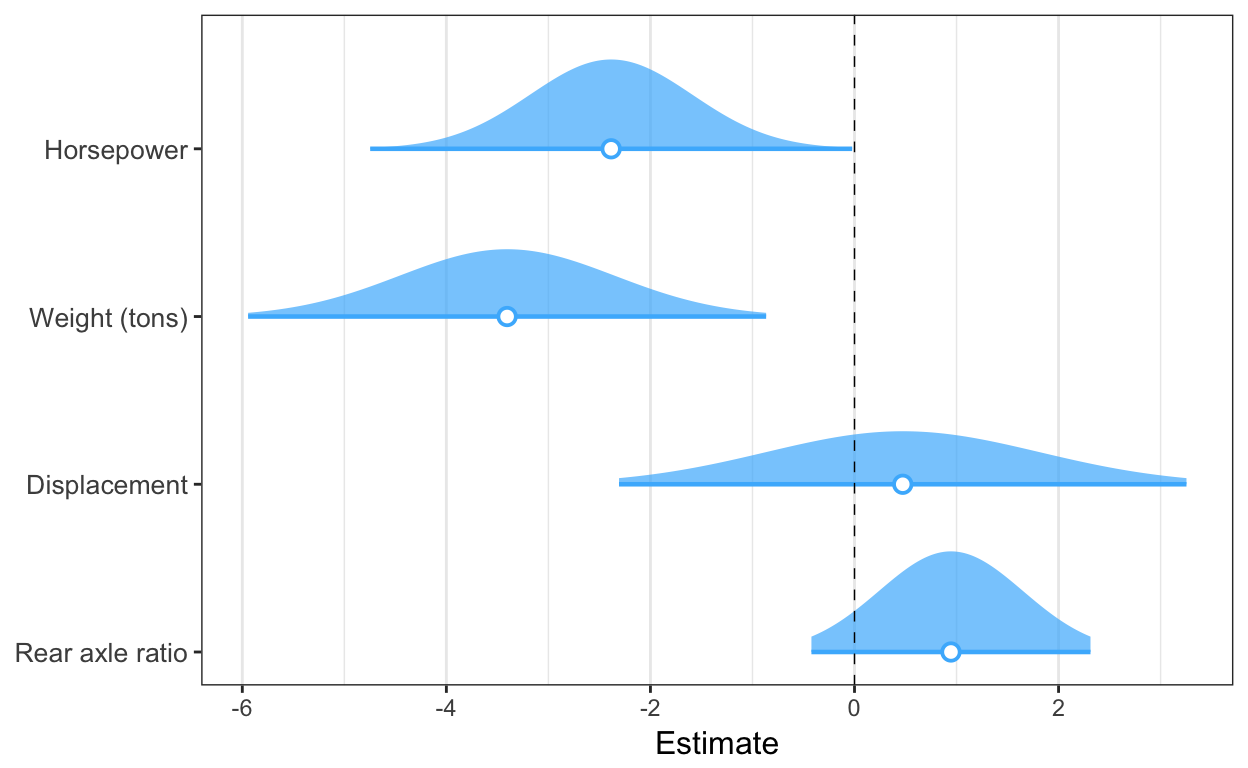These show the 95% interval width of a normal distribution for each estimate.

`plot_coefs` works much the same way, but without support for `summ` arguments like `robust` and `scale`. This enables a wider range of models that have support from the `broom` package but not for `summ`.

### Exploring interactions

Unless you have a really keen eye and good familiarity with both the underlying mathematics and the scale of your variables, it can be very difficult to look at the ouput of regression model that includes an interaction and actually understand what the model is telling you.

This package contains several means of aiding understanding and doing statistical inference with interactions.

#### Johnson-Neyman intervals and simple slopes analysis

The “classic” way of probing an interaction effect is to calculate the slope of the focal predictor at different values of the moderator. When the moderator is binary, this is especially informative—e.g., what is the slope for men vs. women? But you can also arbitrarily choose points for continuous moderators.

With that said, the more statistically rigorous way to explore these effects is to find the Johnson-Neyman interval, which tells you the range of values of the moderator in which the slope of the predictor is significant vs. nonsignificant at a specified alpha level.

The `sim_slopes` function will by default find the Johnson-Neyman interval and tell you the predictor’s slope at specified values of the moderator; by default either both values of binary predictors or the mean and the mean +/- one standard deviation for continuous moderators.

``````fiti <- lm(mpg ~ hp * wt, data = mtcars)
sim_slopes(fiti, pred = hp, modx = wt, jnplot = TRUE)``````
``````#> JOHNSON-NEYMAN INTERVAL
#>
#> When wt is OUTSIDE the interval [3.69, 5.90], the slope of hp is p <
#> .05.
#>
#> Note: The range of observed values of wt is [1.51, 5.42]``````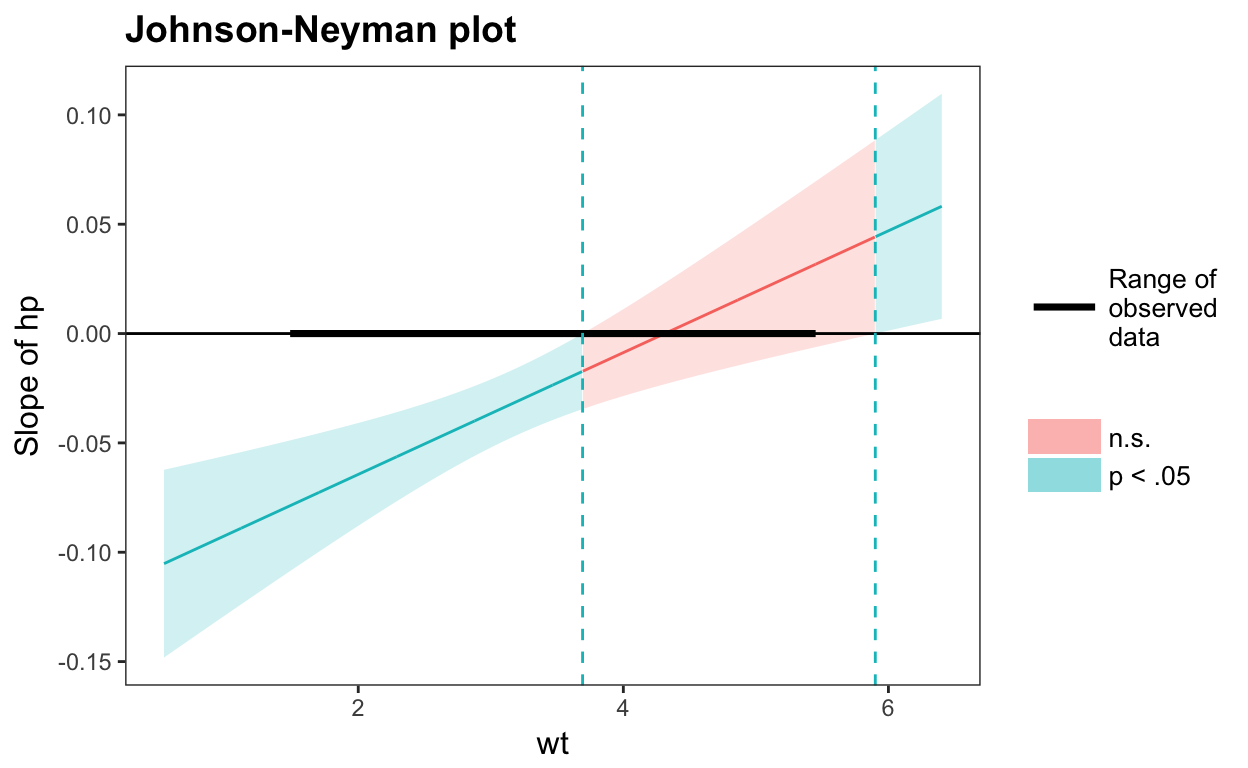``````#> SIMPLE SLOPES ANALYSIS
#>
#> Slope of hp when wt = 4.20 (+ 1 SD):
#>   Est. S.E. t val.    p
#>  -0.00 0.01  -0.31 0.76
#>
#> Slope of hp when wt = 3.22 (Mean):
#>   Est. S.E. t val.    p
#>  -0.03 0.01  -4.07 0.00
#>
#> Slope of hp when wt = 2.24 (- 1 SD):
#>   Est. S.E. t val.    p
#>  -0.06 0.01  -5.66 0.00``````

The Johnson-Neyman plot can really help you get a handle on what the interval is telling you, too. Note that you can look at the Johnson-Neyman interval directly with the `johnson_neyman` function.

The above all generalize to three-way interactions, too.

#### Visualizing interaction effects

This function plots two- and three-way interactions using `ggplot2` with a similar interface to the aforementioned `sim_slopes` function. Users can customize the appearance with familiar `ggplot2` commands. It supports several customizations, like confidence intervals.

``interact_plot(fiti, pred = hp, modx = wt, interval = TRUE)``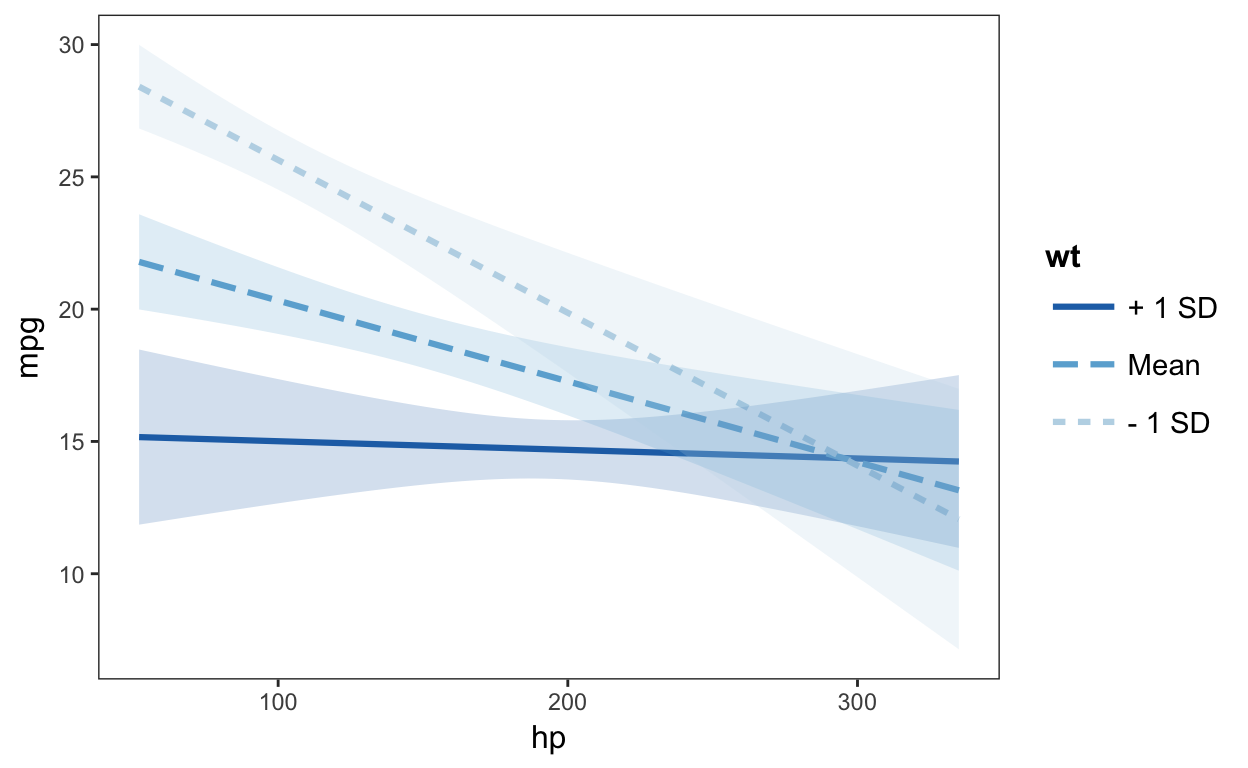You can also plot the observed data for comparison:

``interact_plot(fiti, pred = hp, modx = wt, plot.points = TRUE)``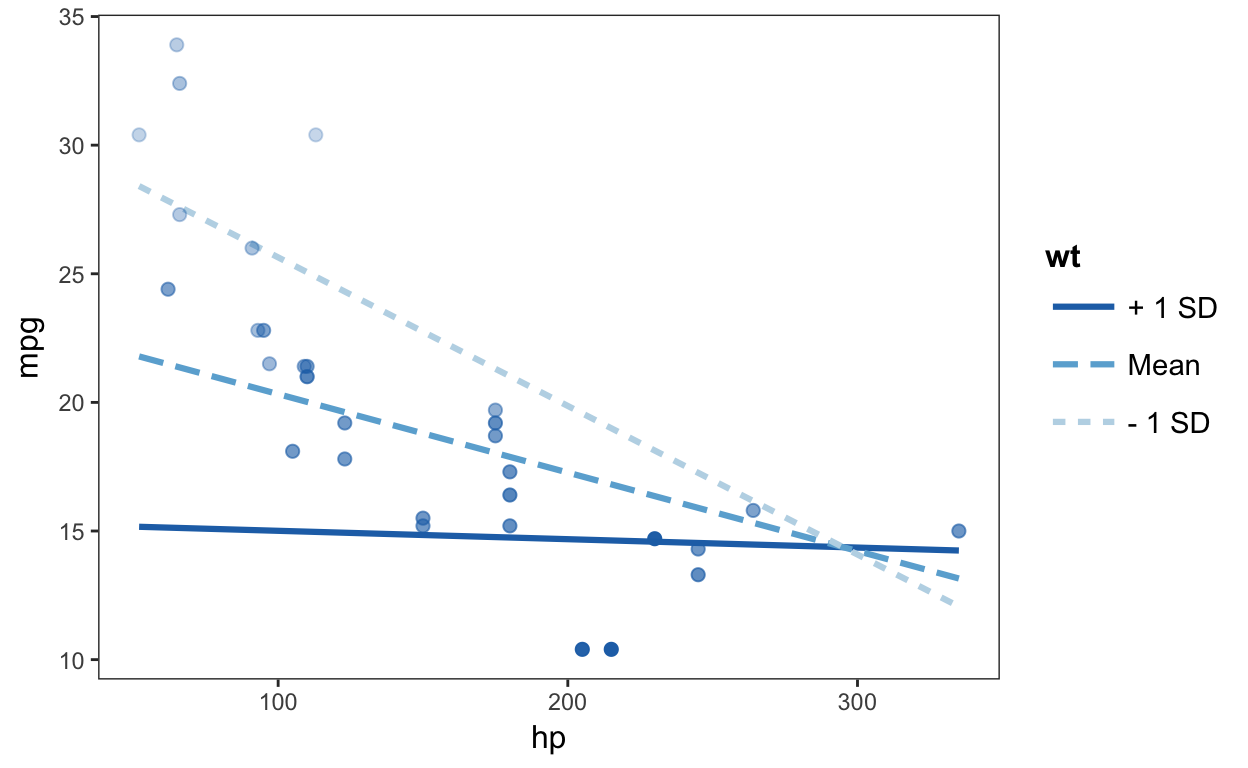The function also supports categorical moderators—plotting observed data in these cases can reveal striking patterns.

``````fitiris <- lm(Petal.Length ~ Petal.Width * Species, data = iris)
interact_plot(fitiris, pred = Petal.Width, modx = Species, plot.points = TRUE)``````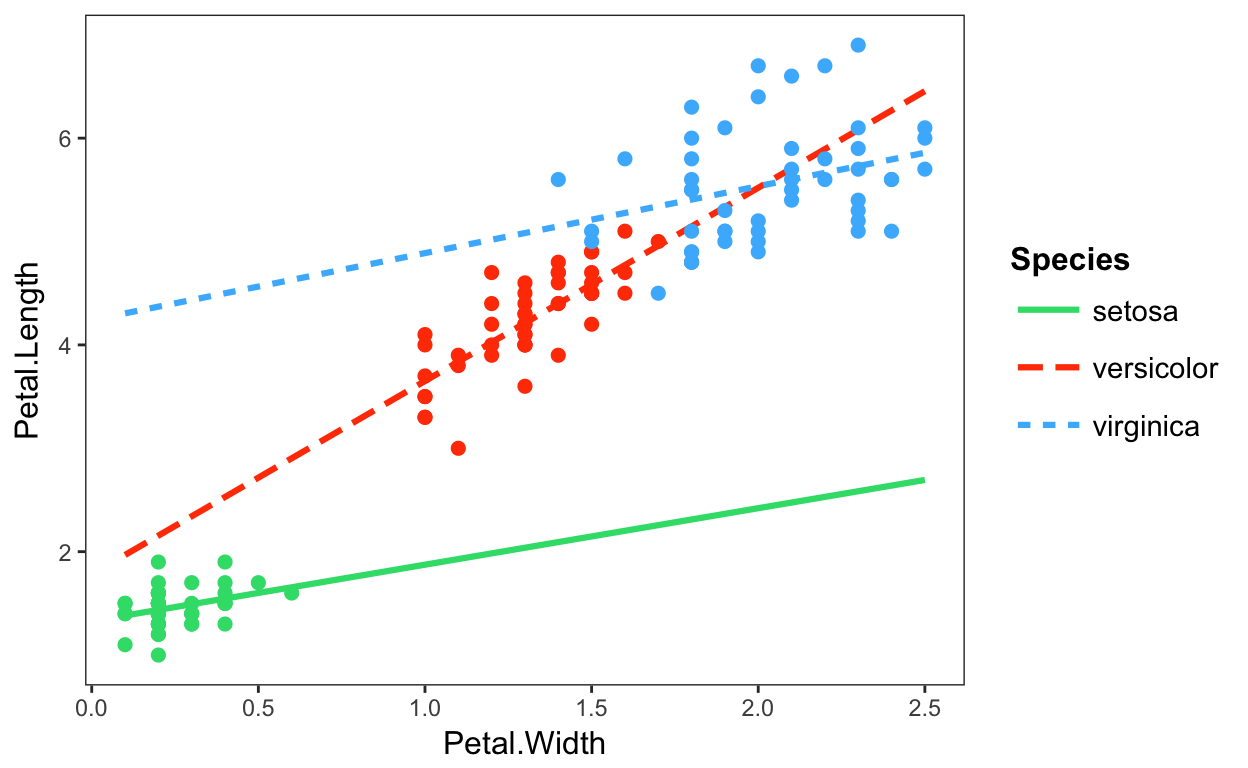You may also combine the plotting and simple slopes functions by using `probe_interaction`, which calls both functions simultaneously. Categorical by categorical interactions can be investigated using the `cat_plot` function.

### Other stuff

There are several other things that might interest you.

• `effect_plot`: Plot predicted lines from regression models without interactions
• `gscale`: Scale and/or mean-center data, including `svydesign` objects
• `scale_mod` and `center_mod`: Re-fit models with scaled and/or mean-centered data
• `wgttest` and `pf_sv_test`, which are combined in `weights_tests`: Test the ignorability of sample weights in regression models
• `svycor`: Generate correlation matrices from `svydesign` objects
• `theme_apa`: A mostly APA-compliant `ggplot2` theme
• `add_gridlines` and `drop_gridlines`: `ggplot2` theme-changing convenience functions
• `make_predictions` and `plot_predictions`: a direct interface to the internals of `interact_plot`, `cat_plot`, and `effect_plot` with some added options

Details on the arguments can be accessed via the R documentation (`?functionname`). There are now vignettes documenting just about everything you can do as well.

## Contributing

I’m happy to receive bug reports, suggestions, questions, and (most of all) contributions to fix problems and add features. I prefer you use the Github issues system over trying to reach out to me in other ways. Pull requests for contributions are encouraged.

Please note that this project is released with a Contributor Code of Conduct. By participating in this project you agree to abide by its terms.